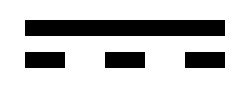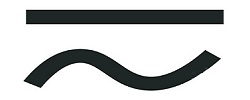The terms we use for electricity and their history Let’s take a look at the terminology used in electronics, where they get their names from and how those terms are used.  The terms used are typically named after famous individuals in the sciences.  Some participated in moving technologies relating to electricity forward while others are named after scientists in their honor for development in other fields Direct Current (DC) –  Direct current is current that flows in one direction.  The earliest example of direct current is Italian physicist Alessandro Volta’s (2/18/1745 – 3/5/1827) battery in 1799.Direct Current symbol Alternating Current (AC) –  Alternating current is an electric current that periodically reverses direction.Alternating Current Symbol Voltage –  Voltage is the difference in electric potential between two points.  It’s essentially the representation of an electrical force or pressure.  To use the age-old analogy of plumbing, voltage would be your water pressure.  We’ll revisit the plumbing analogy later so we can complete our plumbing system as a complete “circuit”. Voltage is represented by the letter “V”, is measured in “volts” and is named after Italian physicist Alessandro Volta.  Volta is credited for inventing the battery in 1799.   A little bit of trivia for you:  Volta also discovered methane (around 1776)! Current – Current in electricity is used in the same way the term “current” is used when talking about the flow of water and air.  Just like how a water flow is water current, electrical current is the rate of flow of electricity. That makes it fairly easy to fit into our plumbing analogy, doesn’t it?  Current IS the water. We measure current in “amperes”, which is named after French physicist André-Marie Ampère (1/20/1775 – 6/10/1836).  The flow of electricity wasn’t discovered by Ampère.  Rather Ampère’s primary work focused specifically on how electrical current influenced magnetic forces.  It was in recognition to Ampère contributions to the sciences that decided, in 1881, that electrical flow be measured in amperes. When defining the amount of current is flowing through a circuit, we tend to use “A” as the designator; but in mathematical equations that include current, we use the letter “I”, which stands for “Intensity”. Wattage – With the definition of wattage, we can create a complete piece of plumbing and try our hand at our first mathematical equation. The symbol for wattage, or watts, is “W” and is named after Scottish inventor James Watt (1/19/1736 – 8/25/1819).  Watt was a mechanical engineer and invented the “Watt Steam Engine” which was a huge improvement over the existing “Newcomen Steam Engine”.  His engine was more efficient, and to show how much more power his engine could produce, developed the concept of “horsepower”.  In his honor, the measurement of power was named after James Watt in 1889. In equations, wattage is represented by the letter “P”, which stands for “power”. In our plumbing analogy, the “wattage” is the amount of water that comes out of the end of our spout.  So let’s build our little hydraulic experiment (not literally). We’ll start with a water tower filled with water.  The water pressure coming down from the tower is our “voltage”.  The rate that the water flows through the pipe from the water tower to the nozzle at the end is “current”, and the amount of water coming out of the spout at the end is our “wattage”. Now here’s the equation for calculating wattage from voltage to current and then we’ll get back to our plumbing project: V * I = P, or:  Voltage times current equals wattage. So 12V at 10A would be 120W.  10V at 12A would also be 120W. In our first plumping example, the water pressure is high as it flows from tower to the spout.  The current is limited by the size of the pipe going from the water tower to the spout.  If we want to increase the “wattage”, what do we do?  We can make the pipe larger!  This increases the current.  The pressure doesn’t change, but the math tells us that wattage will.  You’ll probably notice that with our higher wattage, the tank will drain faster.  This now becomes an analogy for watt-hours…  Watt-hours –  Power, measured in watts, is the rate at which energy is generated or consumed per unit time.  Watt-hours is a measurement of ENERGY, not POWER.  The number of watts used over time (an hour).  Its formula is P (power) * t (time) = E (Energy, or “watt-hours”) For example:  I’ve already told you that I work in the computer industry and my niche in that industry is that I am part of a team that develops power supplies.  If I create a 500W power supply, that’s a power supply that can put out up to 500W.  Watt-hours is how much power is generated by the power supply and/or consumed by the computer. In our plumbing analogy, let’s say our “100W of water” drains the tank in two hours.  With the larger pipe, or higher current, the tank drains in one hour, but we measure the “wattage” of water at 200W.  Either way, the volume of water doesn’t change.  This is shown in the formula for watt-hours.  100 * 2 = 200 and 200 * 1 also equals 200.  We still have the same amount of water… or “energy”.  Coulomb –  Like “watt”, a coulomb is an equation of two other values.  A coulomb is the amount of electrical charge traveling at a constant current per second.  Its symbol is “C” and it is named after French physicist Charles-Augustin de Coulomb (6/14/1736 – 8/23/1806). So our equation for coulomb is, “1C = 1A x 1s”, where “A” is amperage and “s” is second.  One could say that a coulomb is equivalent to “one amp-second”. Joule - One Joule, named after the English physicist James Prescott Joule (12/24/1818 - 10/11/1889) is equal to one watt of power applied for one second. A watt-second, if you will. We use the letter "J" to designate Joules. Joule studied the nature of heat and it's relationship to mechanical work. He developed an apparatus that would actually measure the mechanical equivalent of heat. This was a major contribution to the law of conservation of energy and the first law of thermodynamics. Resistance –  Resistance, as the name implies, defines a material that “resists” the flow of electricity. We measure resistance in “Ohms”.  The term is named after the German physist Georg Simon Ohm (3/16/1789 – 7/6/1854), and the symbol we use for Ohms is the Greek Omega (Ω).  In math equations, we can just use the letter “R” to define resistance, which is much easier than typing ALT+234 on your Windows keyboard. Ω The Greek Omega is used as the symbol for Ohms. Ohm was a professor of math and physics.  Ohm first published what is called “Ohm’s Law” in his 1827 book, “The Galvanic Circuit Investigated Mathematically”.  Using a battery developed by Alessandro Volta, Ohm realized that electric current (I) is proportional to a potential difference, or Voltage (V). To use our plumbing analogy, let’s add a valve to our spout; much like the valve on a garden spout.  As you tighten the valve, it creates resistance that lowers the water pressure.  Water pressure, of course, being the equivalent of “voltage”. To calculate the impact of resistance on electrical current, we use the equation of I = V / R.  And, as we learned in first year algebra, likewise you can calculate resistance’s impact on voltage, we can use V = I * R.  Finally, resistance would be R = V / I. Let’s say we have 100V of potential energy and 2A of current and we want to “solve for R”.  R = 100 / 2, or 50Ω. In practical application, resistors create resistance to reduce current or voltage.  So we’ll be using Ohm’s math and some variations of it in the near future. Hertz – Hertz is a measuring unit for measuring the number of cycles per second.  Hertz is used to measure the waveforms of sounds and other signals, but initially it was named for the realization that electromagnetic waves existed.  This discovery was made by Heinrich Hertz (2/22/1857 – 1/1/1894), which is where the term comes from.  Hertz can be used to measure how many cycles alternating current alternates within one second.  In the US, for example, alternating current alternates at a frequency of 60Hz, or 60 cycles.  That means it alternates once every 16.666 milliseconds, because we can take 1000 and divide by 60.  In the EU, AC has a frequency of 50Hz.  So if we take 1000 (for 1000 cycles per second) and divide by 50 (50 cycles), each cycle lasts 20ms.     Capacitance – Capacitance is a component’s ability to collect and store energy. Probably the most common component associated with this term is the “capacitor”, which is a component used to store energy in a circuit. Capacitance is measured in “Farads”, represented by the letter “F”, and is named after English scientist Michael Faraday. Inductance –  The term “Inductance” was first coined by English engineer Oliver Heaviside (5/18/1850 – 2/3/1925).  And while Heaviside did not “discover electromagnetic induction, as that credit goes to Michael Faraday, Heaviside is credited for giving it a name.  On paper, we use the letter "L" as the symbol for Inductance. This is in honour of Heinrich Emil Lenz (2/12/1804 - 2/10/1865), who formulated "Lenz's Law" which states that "The current induced in a circuit due to a change in a magnetic field is directed to oppose the change in flux and to exert a mechanical force which opposes the motion." We’ll get to talk about another one of Heaviside’s terms, “impedance”, in a bit. Reactance – Reactance occurs with alternating current.  Reactance is similar to resistance.  When alternating current passes through a circuit, the phase and amplitude of current will change.  This creates energy released in the form of a magnetic field. In equations, you would use "X" to define reactance. But reactance is measured in Ohms. Impedance –  Impedance is another one of Heaviside’s coined terms.  So, what is impedance?  One measures the opposition applied to current when voltage is applied to a circuit as impedance.  “Wait a minute!  Isn’t current’s impact on voltage called ‘resistance’??”  Yes.  But there is a bit of a difference here. First off, while resistance can occur in either AC or DC circuits, impedance only occurs in AC circuits.  Furthermore, impedance occurs as a result of resistance, capacitance and inductance, which is why I put its definition at the end here.  Remember that AC “alternates”.  Since the power oscillates as a sine wave, these other things need to be taken into consideration. In equations, you would use "Z" to define impedance. But impedance is measured in Ohms.